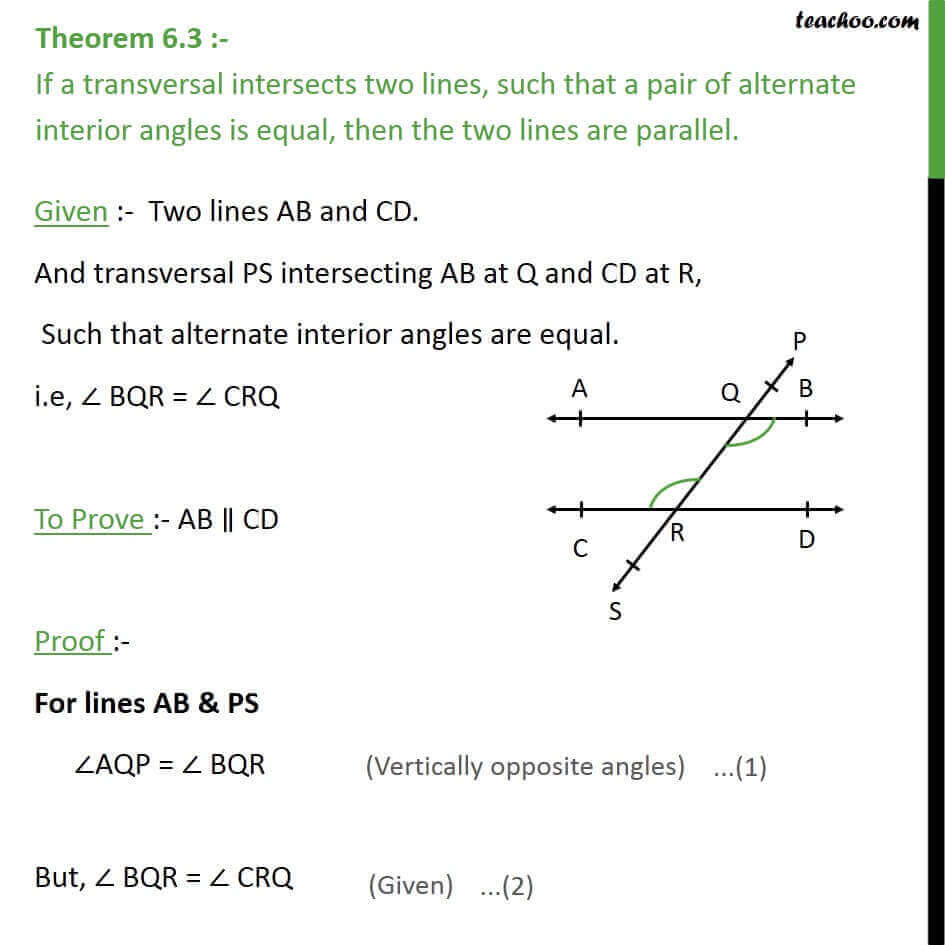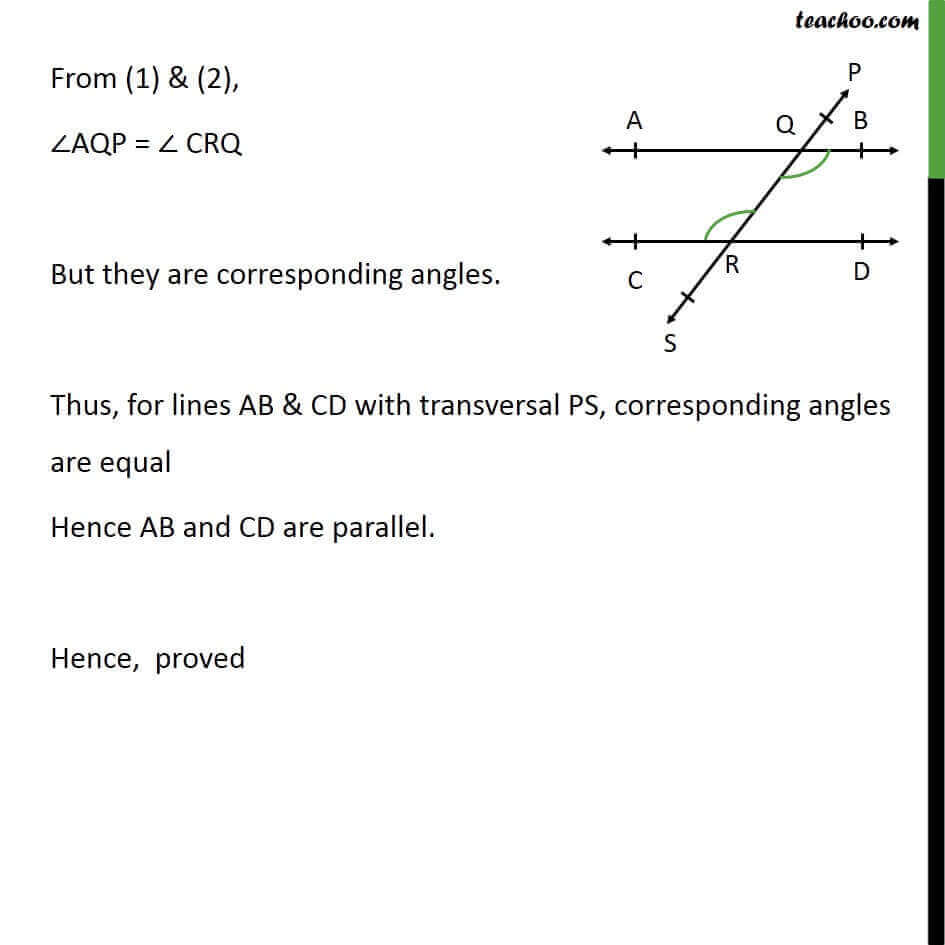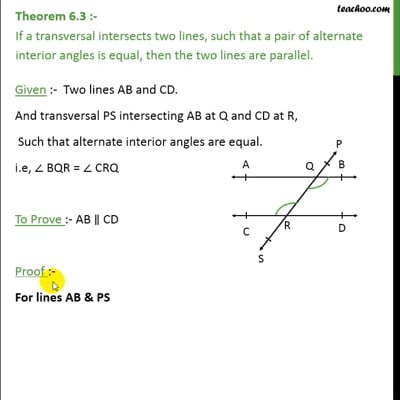Theorems

Chapter 6 Class 9 Lines and Angles (Term 1)
Serial order wiseThis video is only available for Teachoo black users

### Transcript

Theorem 6.3 :- If a transversal intersects two lines, such that a pair of alternate interior angles is equal, then the two lines are parallel. Given :- Two lines AB and CD. And transversal PS intersecting AB at Q and CD at R, Such that alternate interior angles are equal. i.e, BQR = CRQ To Prove :- AB CD Proof :- For lines AB & PS AQP = BQR But, BQR = CRQ From (1) & (2), AQP = CRQ But they are corresponding angles. Thus, for lines AB & CD with transversal PS, corresponding angles are equal Hence AB and CD are parallel. Hence, proved